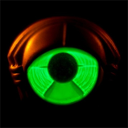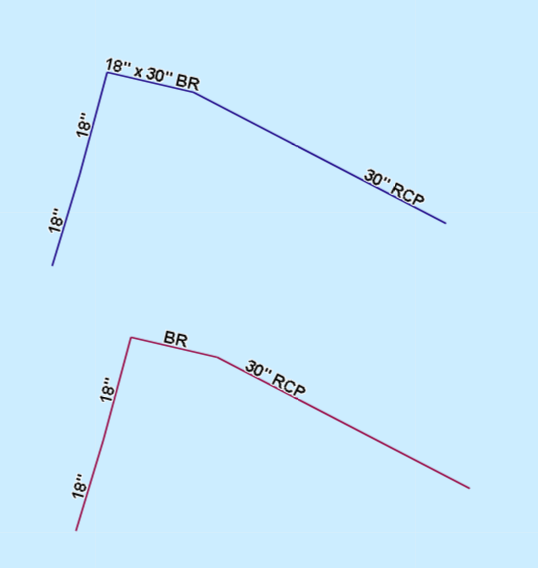# Why isn't my Arcade expression returning a label in ArcGIS online (despite testing successfully)?

642
4
05-21-2019 04:37 AMby
Regular Contributor

I wrote the label expression below to look at pipe length and cross section shape to determine if and how a label is generated for a pipe. It works fine in ArcGIS Pro, and when I paste the expression into ArcGIS Online the code validates and returns a test string as expected (for both label functions in the expression).

However, no labels are displaying on the map. I have a similar (and more simple) function that is working just fine.

``````function ChooseLabelFormat(ML,PL,Sec){
//Calls the label function based on pipe shape
return When(
PL >= ML && Sec == 'Circular', GetMainLabel (\$feature.NominalDiameter,\$feature.Material),
PL >= ML && Sec != 'Circular', GetMainLabelNC (\$feature.Material,\$feature.BoxHeight,\$feature.BoxWidth),
"")
};

function GetMainLabel(Dia,Mat){
//Returns Diameter and/or material excluding -999 and Unk values
return When(
Dia !=-999 && Mat != "Unk", Dia + "'' " + Mat,
Dia !=-999 && Mat == "Unk", Dia + "''",
Dia ==-999 && Mat != "Unk", Mat,
"")
};

function GetMainLabelNC(Mat,BH,BW){
//Returns HeightxWidth and/or material excluding -999 and Unk values
return When(
BH !=-999 && Mat != "Unk", BH + "'' x " + BW + "'' " + Mat,
BH !=-999 && Mat == "Unk", BH + "'' x " + BW + "'' ",
BH ==-999 && Mat != "Unk", Mat,
"")
};

//Set variables and call function for labeleling
var Section = DomainName(\$feature, 'CrossSectionShape');
// Set MinLabel value as the minimum feature length to be labeled:
var MinLabel = 50;
var PipeLen = \$feature.SHAPE__Length; //var PipeLen = Length(Geometry(\$feature), 'feet')

return ChooseLabelFormat(MinLabel,PipeLen,Section);‍‍‍‍‍‍‍‍‍‍‍‍‍‍‍‍‍‍‍‍‍‍‍‍‍‍‍‍‍‍‍‍‍‍‍‍‍‍‍‍‍‍‍‍‍‍‍‍‍‍‍‍‍‍‍‍‍‍‍‍‍‍‍‍‍‍``````
Tags (2)
4 RepliesbyEsri Esteemed Contributor

Can you provide some test values for MinLabel, PipeLen and Section? Or much beter,  access to the data? It would be good to see a screen shot from the same area in Pro (with the labels) and ArcGIS Online (where they fail).

Edit: I noticed at the end of your expression that you commented the PipeLen expression calculated in feet. Are you sure that the PipeLen is now returned in feet. In ArcGIS Online Web Mercator Auxiliary Sphere is used.by
Regular Contributor

Thanks Xander Bakker

See example here: http://arcg.is/1Tzjiq

There is one layer with the code in my original post, which does not produce a label.

There is a second layer with the code shown below that does produce a label.

``````//Returns Diameter and/or material excluding -999 and Unk values
function GetMainLabel(Dia,Mat) {
return When(Dia !=-999 && Mat != "Unk", Dia + "'' " + Mat, Dia !=-999 && Mat == "Unk", Dia + "''", Dia ==-999 && Mat != "Unk", Mat,"")
}

// Set MinLabel value as the minimum feature length to be labeled:
var MinLabel = 50;
var PipeLen = \$feature.SHAPE__Length; //var PipeLen = Length(Geometry(\$feature), 'feet');
iif (PipeLen >= MinLabel, GetMainLabel (\$feature.NominalDiameter,\$feature.Material),'');‍‍‍‍‍‍‍‍‍``````

Regarding the length, that's actually commented out and the length is taken from Shape__Length. However, the data was in NJ State Plane when published, and it's my understanding that it stays that way and gets projected on the fly in AGOL. Is this not correct? The spatial reference WKID is 102711 (NJ state plane NAD83)per the json (see screen shot)byEsri Esteemed Contributor

That is indeed very strange behavior. When I use the label expression and use it in the pop-up, it validates correctly with every feature. However, it does not return something in the pop-up or label. Very strange. I tried to rewrite the code and this resulted in the same behavior.

``````function ChooseLabelFormat(ML,PL,Sec){
//Calls the label function based on pipe shape
if (PL >= ML) {
if (Sec == 'Circular') {
return GetMainLabel(\$feature.NominalDiameter,\$feature.Material);
} else {
return GetMainLabelNC(\$feature.Material,\$feature.BoxHeight,\$feature.BoxWidth);
}
} else {
return "";
}
}

function GetMainLabel(Dia,Mat){
//Returns Diameter and/or material excluding -999 and Unk values
if (Dia !=-999) {
if (Mat != "Unk") {
return Dia + "'' " + Mat;
} else {
return Dia + "''";
}
} else {
if (Mat != "Unk") {
return Mat;
} else {
return "";
}
}
}

function GetMainLabelNC(Mat,BH,BW){
//Returns HeightxWidth and/or material excluding -999 and Unk values
if (BH !=-999) {
if (Mat != "Unk") {
return BH + "'' x " + BW + "'' " + Mat;
} else {
return BH + "'' x " + BW + "'' ";
}
} else {
if (Mat != "Unk") {
return Mat;
} else {
return "";
}
}
}

//Set variables and call function for labeleling
var Section = DomainName(\$feature, 'CrossSectionShape');
// Set MinLabel value as the minimum feature length to be labeled:
var MinLabel = 50;
var PipeLen = \$feature.SHAPE__Length; //var PipeLen = Length(Geometry(\$feature), 'feet')

return ChooseLabelFormat(MinLabel,PipeLen,Section);‍‍‍‍‍‍‍‍‍‍‍‍‍‍‍‍‍‍‍‍‍‍‍‍‍‍‍‍‍‍‍‍‍‍‍‍‍‍‍‍‍‍‍‍‍‍‍‍‍‍‍‍‍‍``````

Maybe something for support?

If I retrieve the WKID of the geometry it will return 102100 (Web Mercator Auxiliary Sphere):

``````return \$feature.OBJECTID;
// returns 306

return Geometry(\$feature).spatialReference["wkid"];
// returns 102100 which is (Web Mercator Auxiliary Sphere)

return Length(\$feature, "meter");
// returns 22.8405....

return Length(\$feature, "feet");
// returns 74.9362....

return \$feature["Shape__Length"];
// returns 57.2223....

return LengthGeodetic(\$feature, "meter");
// returns 17.4116....

return LengthGeodetic(\$feature, "feet");
// returns 57.1249....
‍‍‍‍‍‍‍‍‍‍‍‍‍‍‍‍‍‍‍‍``````

So the Shape__Length represents the length in feet (Geodetic).

I will try one last thing to see if it works...byEsri Esteemed Contributor

OK, so for some reason it does not like the functions (I guess). If I use this expression it seems to work:

``````var Sec = DomainName(\$feature, 'CrossSectionShape');
var ML = 50;
var PL = \$feature.SHAPE__Length;
var Dia = \$feature.NominalDiameter;
var Mat = \$feature.Material;
var BH = \$feature.BoxHeight;
var BW = \$feature.BoxWidth;

var GetMainLabel = When(
Dia !=-999 && Mat != "Unk", Dia + "'' " + Mat,
Dia !=-999 && Mat == "Unk", Dia + "''",
Dia ==-999 && Mat != "Unk", Mat,
"");

var GetMainLabelNC = When(
BH !=-999 && Mat != "Unk", BH + "'' x " + BW + "'' " + Mat,
BH !=-999 && Mat == "Unk", BH + "'' x " + BW + "'' ",
BH ==-999 && Mat != "Unk", Mat,
"");

var result = When(
PL >= ML && Sec == 'Circular', GetMainLabel,
PL >= ML && Sec != 'Circular', GetMainLabelNC,
"");

return result;‍‍‍‍‍‍‍‍‍‍‍‍‍‍‍‍‍‍‍‍‍‍‍‍‍‍``````